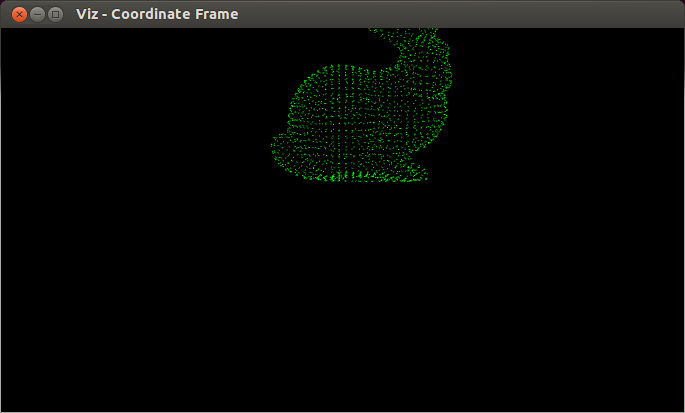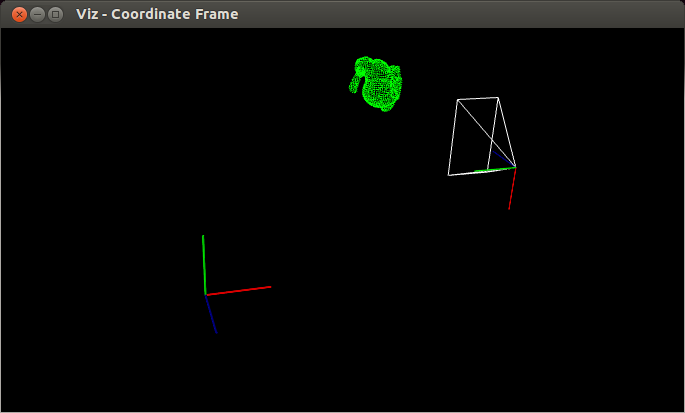# Transformations¶

## Goal¶

In this tutorial you will learn how to

• How to use makeTransformToGlobal to compute pose
• How to use makeCameraPose and Viz3d::setViewerPose
• How to visualize camera position by axes and by viewing frustum

## Code¶

You can download the code from `here`.

```#include <opencv2/viz/vizcore.hpp>
#include <iostream>
#include <fstream>

using namespace cv;
using namespace std;

/**
*/
{
Mat cloud(1, 1889, CV_32FC3);
ifstream ifs("bunny.ply");

string str;
for(size_t i = 0; i < 12; ++i)
getline(ifs, str);

Point3f* data = cloud.ptr<cv::Point3f>();
float dummy1, dummy2;
for(size_t i = 0; i < 1889; ++i)
ifs >> data[i].x >> data[i].y >> data[i].z >> dummy1 >> dummy2;

cloud *= 5.0f;
return cloud;
}

/**
* @function main
*/
int main(int argn, char **argv)
{
if (argn < 2)
{
cout << "Usage: " << endl << "./transformations [ G | C ]" << endl;
return 1;
}

bool camera_pov = (argv == 'C');

/// Create a window
viz::Viz3d myWindow("Coordinate Frame");

myWindow.showWidget("Coordinate Widget", viz::WCoordinateSystem());

/// Let's assume camera has the following properties
Point3f cam_pos(3.0f,3.0f,3.0f), cam_focal_point(3.0f,3.0f,2.0f), cam_y_dir(-1.0f,0.0f,0.0f);

/// We can get the pose of the cam using makeCameraPose
Affine3f cam_pose = viz::makeCameraPose(cam_pos, cam_focal_point, cam_y_dir);

/// We can get the transformation matrix from camera coordinate system to global using
/// - makeTransformToGlobal. We need the axes of the camera
Affine3f transform = viz::makeTransformToGlobal(Vec3f(0.0f,-1.0f,0.0f), Vec3f(-1.0f,0.0f,0.0f), Vec3f(0.0f,0.0f,-1.0f), cam_pos);

/// Create a cloud widget.
viz::WCloud cloud_widget(bunny_cloud, viz::Color::green());

/// Pose of the widget in camera frame
Affine3f cloud_pose = Affine3f().translate(Vec3f(0.0f,0.0f,3.0f));
/// Pose of the widget in global frame
Affine3f cloud_pose_global = transform * cloud_pose;

/// Visualize camera frame
if (!camera_pov)
{
viz::WCameraPosition cpw(0.5); // Coordinate axes
viz::WCameraPosition cpw_frustum(Vec2f(0.889484, 0.523599)); // Camera frustum
myWindow.showWidget("CPW", cpw, cam_pose);
myWindow.showWidget("CPW_FRUSTUM", cpw_frustum, cam_pose);
}

/// Visualize widget
myWindow.showWidget("bunny", cloud_widget, cloud_pose_global);

/// Set the viewer pose to that of camera
if (camera_pov)
myWindow.setViewerPose(cam_pose);

/// Start event loop.
myWindow.spin();

return 0;
}
```

## Explanation¶

Here is the general structure of the program:

• Create a visualization window.
```/// Create a window
viz::Viz3d myWindow("Transformations");
```
• Get camera pose from camera position, camera focal point and y direction.
```/// Let's assume camera has the following properties
Point3f cam_pos(3.0f,3.0f,3.0f), cam_focal_point(3.0f,3.0f,2.0f), cam_y_dir(-1.0f,0.0f,0.0f);

/// We can get the pose of the cam using makeCameraPose
Affine3f cam_pose = viz::makeCameraPose(cam_pos, cam_focal_point, cam_y_dir);
```
• Obtain transform matrix knowing the axes of camera coordinate system.
```/// We can get the transformation matrix from camera coordinate system to global using
/// - makeTransformToGlobal. We need the axes of the camera
Affine3f transform = viz::makeTransformToGlobal(Vec3f(0.0f,-1.0f,0.0f), Vec3f(-1.0f,0.0f,0.0f), Vec3f(0.0f,0.0f,-1.0f), cam_pos);
```
• Create a cloud widget from bunny.ply file
```/// Create a cloud widget.
viz::WCloud cloud_widget(bunny_cloud, viz::Color::green());
```
• Given the pose in camera coordinate system, estimate the global pose.
```/// Pose of the widget in camera frame
Affine3f cloud_pose = Affine3f().translate(Vec3f(0.0f,0.0f,3.0f));
/// Pose of the widget in global frame
Affine3f cloud_pose_global = transform * cloud_pose;
```
• If the view point is set to be global, visualize camera coordinate frame and viewing frustum.
```/// Visualize camera frame
if (!camera_pov)
{
viz::WCameraPosition cpw(0.5); // Coordinate axes
viz::WCameraPosition cpw_frustum(Vec2f(0.889484, 0.523599)); // Camera frustum
myWindow.showWidget("CPW", cpw, cam_pose);
myWindow.showWidget("CPW_FRUSTUM", cpw_frustum, cam_pose);
}
```
• Visualize the cloud widget with the estimated global pose
```/// Visualize widget
myWindow.showWidget("bunny", cloud_widget, cloud_pose_global);
```
• If the view point is set to be camera’s, set viewer pose to cam_pose.
```/// Set the viewer pose to that of camera
if (camera_pov)
myWindow.setViewerPose(cam_pose);
```

## Results¶

1. Here is the result from the camera point of view.2. Here is the result from global point of view.